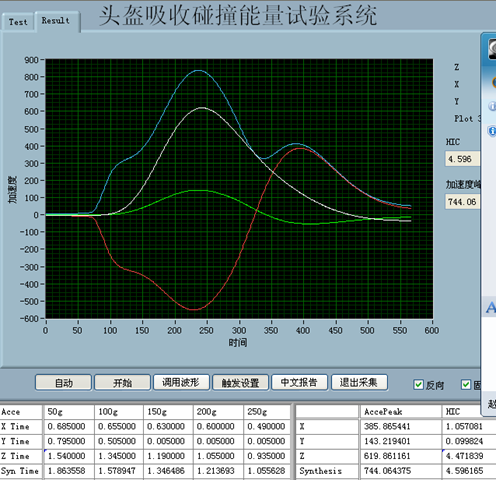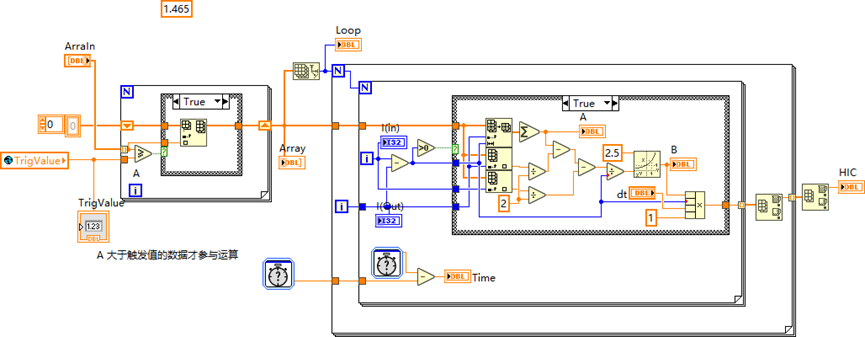# LabVIEW编程LabVIEW开发NI USB 6251例程与相关资料LabVIEW编程LabVIEW开发NI USB 6251例程与相关资料

USB-6251提供模拟I/O、数字I/O、32计数器/数字触发，用于头盔吸收碰撞能量试验的一个项目。E = 0                                                                              'E作为放最大HIC值

T = 0.05                                                                            '定义采样的时间间隔,单位ms

C =Application.WorksheetFunction.Count(Range("A:A"))                               '获取A列数据(即试验数据)的个数,作为循环的作大数

For i = 1 To C                                                                     '对t1采样点由起始数据到末尾数据的遍历

For j = (i + 1) To C                                                           '对t2采样点由t1后加1个数据到末尾数据的遍历

A =Application.WorksheetFunction.Sum(Range(Cells(i, 1), Cells(j, 1)))          '对t1采样点到t2采样点加速度值的求和

'Cells(j, 7 + i) = A   '显示A值计算过程,用于调试

B = ((A - Cells(i, 1) / 2 - Cells(j, 1) /2) / (j - i)) ^ 2.5                   '对HIC公式2.5次幂内的计算

HIC = B * (j - i) * T * 0.001                                                  '对HIC公式的计算(*0.001,因为时间的单位市ms,所以*0.001)

'Cells(j, 7 + i) = HIC   '显示HIC值计算过程,用于调试

If HIC > E Then

E = HIC                                                                   '找最大的HIC值,并赋值给变量E

Else

End If

Next j

Next i

Range("b1").Value = E                                                           '将最大HIC值显示在b1单元格

End Sub13691203761王工（手机微信）